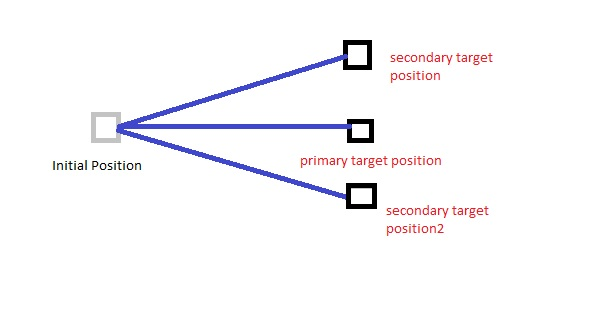Hi, my first question, maybe a very noob question but I need some help …

let say that I want to shoot 3 bullets in different directions.

Please refer the img for reference.Actually I´m using 3 diferent objects as targets, but I would like to have only one target and use angles to set the direction of the other 2.

this is what I’m using to get the direction:

``````fireDirection = (targetPosition - originPosition).normalized;
``````

How can I add n degrees to my fireDirection?

I ended up with some trigonometry, thanks alot for all your help, you guys guided me to the solution.

here’s the code, I’ve tested it with Debug.DrawLine

``````Vector3[] CalculatePositionsForTripleDirection(){
Vector3[] positions = new Vector3;

float r = Vector3.Distance(bulletOriginPosition,targetPosition);
float x = Mathf.Abs(bulletOriginPosition.x - targetPosition.x);
float y = Mathf.Abs(bulletOriginPosition.y - targetPosition.y);
float initialAngle = Mathf.Atan2(y,x) * Mathf.Rad2Deg;
float secondAngle = (initialAngle + angleVariance) * Mathf.Deg2Rad;
float thirdAngle = (initialAngle - angleVariance) * Mathf.Deg2Rad;

//Calculate x2 and y2
float x2 = r  * Mathf.Cos(secondAngle);
float y2 = r  * Mathf.Sin(secondAngle);

//Calculate x3 and y3
float x3 = r  * Mathf.Cos(thirdAngle);
float y3 = r  * Mathf.Sin(thirdAngle);

//Verify if X is positive or negative
if(targetPosition.x <  bulletOriginPosition.x){
x2 = x2 *-1;
x3 = x3 *-1;
}

//Verify if Y is positive or negative
if(targetPosition.y < bulletOriginPosition.y){
y2 = y2 * -1;
y3 = y3 * -1;
}

//Assign Values to positions
positions = targetPosition;
positions = new Vector3(bulletOriginPosition.x + x2, bulletOriginPosition.y + y2,targetPosition.z);
positions = new Vector3(bulletOriginPosition.x + x3,bulletOriginPosition.y + y3,targetPosition.z);
return positions;
}
``````